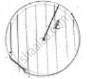Share

# A Horse is Tied to a Pole with 28m Long String. Find the Area Where the Horse Can Graze. - CBSE Class 10 - Mathematics

ConceptPerimeter and Area of a Circle

#### Question

A horse is tied to a pole with 28m long string. Find the area where the horse can graze.

#### Solution

Length of string 1 = 28mArea it can graze is area of circle with radius equal to length of string

Area = 𝜋l2

= 22/7 × 28 × 28

= 88 × 28

= 2464 cm2

∴ area grazed by horse = 2464cm2.

Is there an error in this question or solution?

#### Video TutorialsVIEW ALL 

Solution A Horse is Tied to a Pole with 28m Long String. Find the Area Where the Horse Can Graze. Concept: Perimeter and Area of a Circle.
S